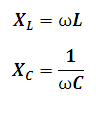# Reactance Calculator

This Calctown Calculator calculates the inductive and capacitive reactance magnitude of a given circuit.

H
F
Hz

#### Result

ohm
ohmwhere

L = inductance

C = capacitance

XL = inductive reactance

XC = capacitive reactance

f = frequency

ω = angular frquency